Select Page

# Turing Machine With Example

A Turing Machine can be explained through 7-Tuples (Q, T, B, ∑, δ, q0, F). These 7-Tuples with example are explain below

7-Tuples are

• Q is a finite set of states
• T is the input tape which holds the Input and Special symbols. So, Both input and Special symbols are subset of input tape T.
• B is Special symbol also called Blank symbol (every cell is filled with special symbol except input alphabet initially)
• is the input symbols (input tape holds initially input alphabet)
• δ is a transition function is Q × T → Q × T × {L,R}. At any state (Q) when the
• Input tape symbol (T) is applied then  it will move to new state, Update the tape symbol (may or may not change)  and move head pointer to either right or left.
• q0 is the initial state.
• F is the set of final states. If any state of F is reached, input string is accepted.

## Example of Turing Machine

Let us construct a Turing machine for very basic language L= “010”. The Input tape for language “010” will become as follows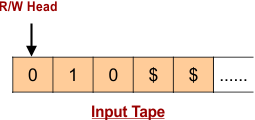After placing given language “010” In the input tape, special symbols are inserted. For the arrival of first special symbol the given language is accepted.

Consider,

• Q = {q0,q1,q2,q3} where q0 is initial state.
• T = {0,1,X,Y,\$} where \$ represents blank.
• ∑ = {0,1}
• F = {q3}
 Important: Symbols “X and Y” In input Tape are used to Update values of Sigma input ∑ (0,1). It is just identification which tells that the input symbols are read successfully. (i.e. when read Sigma input “0” it should update with “X” in input-tap and “1” use “Y”).

### For First Input “0”

• Transition goes to state (q1) from initial state (q0)
• The first symbol “0” of input tape is updated to “X”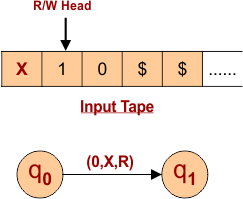### For Second Input “1”

• Transition goes to state (q2) from state (q1)
• The second symbol “1” of input tape is updated to “Y”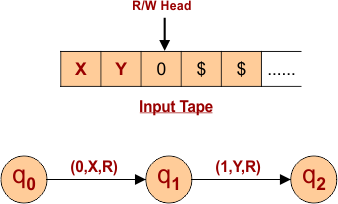### For Third Input “0”

• Transition goes to state (q3) from state (q2)
• The third symbol “0” of input tape is updated to “X”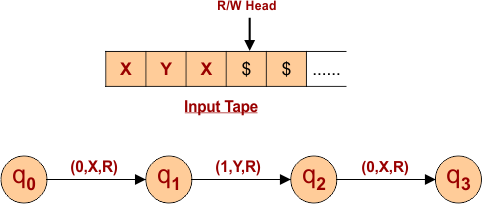### For First Special Symbol “\$”

• Transition goes to state (q4) from state (q3) which is accepting state.
• The special symbol “\$” of input tape unchanged and remain same as “\$”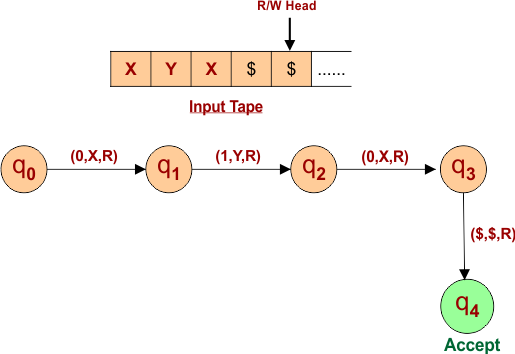Other than “010” language all language are rejected. All possible path for each input (0,1 and \$) at each state is given below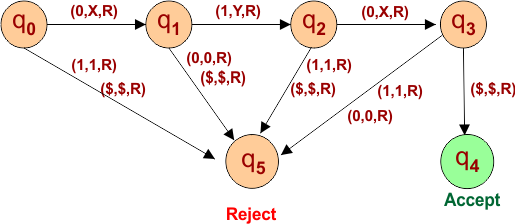Help Other’s By Sharing…